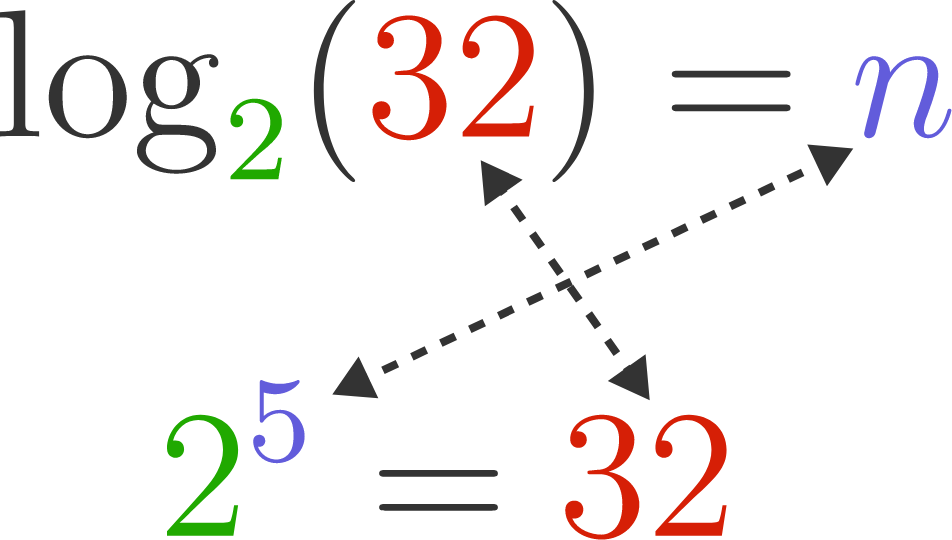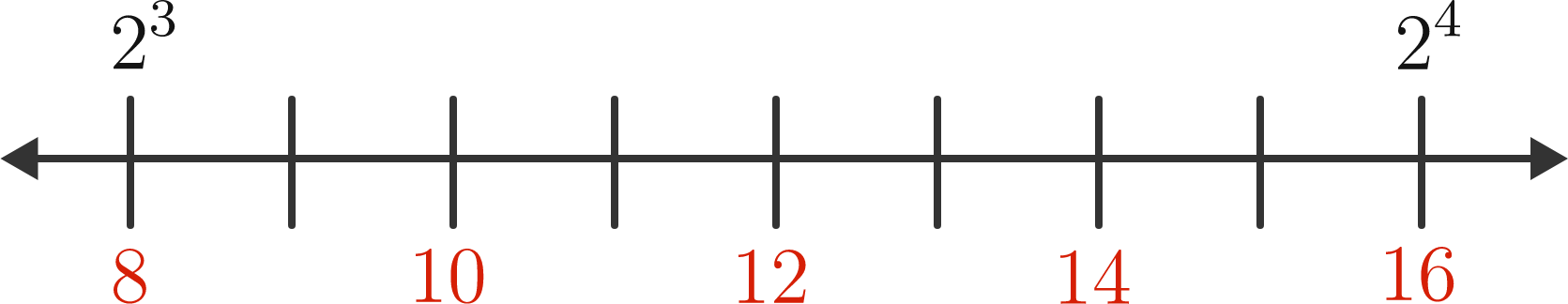### Pre-Calculus

$2^n = 3.91 \times 10^{12}$

Given some equation like the one above, with a base and the result after raising it to a power, how do we find the power that was used? Essentially, we are taking the inverse of the exponential function. This inverse function is a logarithm written as "log".Calculating the logarithm $\log_2(32)$ is akin to asking: $2$ raised to what power is $32?$ Since $2^5 = 32 ,$ $\log_2(32) = 5 .$

What is $\log_6(36) ?$

# Logarithms

Which of these two numbers is larger?

$\log_{10}(1000) \quad \text{ and } \quad \log_2(9)$

Remember, you can read $\log_b(x)$ as "$b$ raised to what power is $x ?$"

# Logarithms

Since exponents don't need to be integers...

$4^{3.5} = 128$

...the results of logarithms don't need to be either!

$\log_{4}(128) = 3.5$

We can think of the logarithms that come out to be integers as "reference points".

$\log_{2}{2} = 1 \quad \log_{2}{4} = 2 \quad \log_{2}{8} = 3 \quad \log_{2}{16} = 4$

$\log_2{7}$ is...

# Logarithms

$\log_{10}(1000) = 3$

$\log_{10}(49323) \approx 4.69$

$\log_{10}(333333) \approx 5.52$

$\log_{10}(100000) = 5$

Which statement is true? $($Assume you are working with numbers in base $10.)$

# Logarithms$\log_2(12)$ is...

# Logarithms

We're going to finish our tour of exponential and logarithm functions by going back into space on a journey to the edge of the universe. We'll use something called a logarithmic scale to help us out, and establish the framework for the mathematics that starts our Pre-Calculus course. Read on!×